C Program to Round off Floating point Number

In this tutorial you will learn about the C Program to Round off Floating point Number and its application with practical example.

C Program to Round off Floating point Number

In this tutorial, we will learn to create a C program that will Round off Floating-point Numbers in C programming.

Prerequisites

Before starting with this tutorial we assume that you are best aware of the following C programming topics:

• Operators in C Programming.
• Basic Input and Output function in C Programming.
• Basic C programming.
• Conditional Statements in c programming.

Round off Floating point Number.

As we all know the c programming is a very powerful language. In the c programming language, we have many pre-defined functions for arithmetic operations. But today we will Round off the Floating-point Numbers using the c programming language.

With C programming we can make many arithmetics series with just a little code.

With the help of this program, we can Round off Floating-point Numbers.

Output:-

Positive round-of:-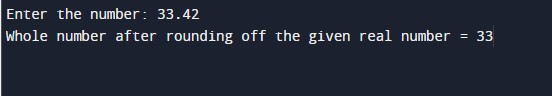Negative round-of:-In the above program, we have first initialized the required variable.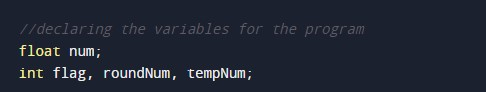• num = it will hold the floating number value.
• flag = it will hold the integer value.
• roundNum = it will hold the integer value.
• tempNum = it will hold the integer value.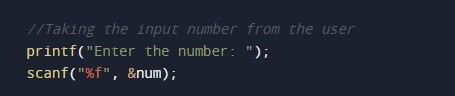Checking the number greater than zero.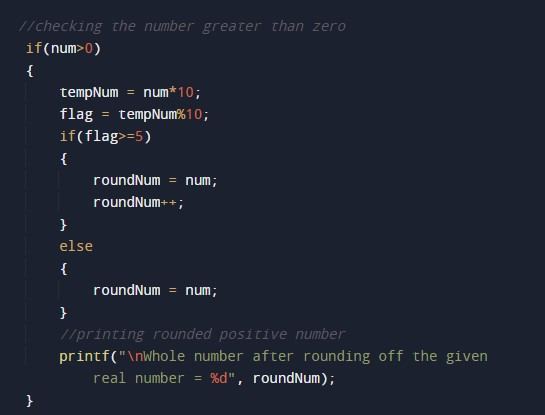Checking the number smaller than zero.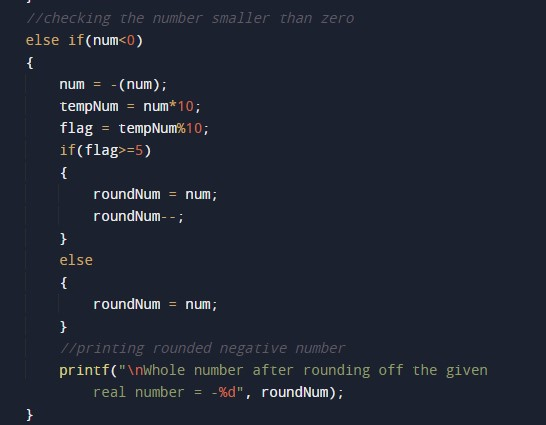Printing the number equal to zero.In this tutorial we have learn about the C Program to Round off Floating point Number and its application with practical example. I hope you will like this tutorial.